# 4.NF.5 & 4.NF.6 Fractions and Decimals

##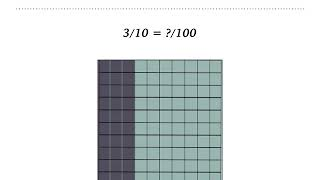By Tiffany Nickel

an understanding of fraction equivalence with denominators 10 and 100 as well as decimal notation.# Relevance: Use of Irrational Numbers

##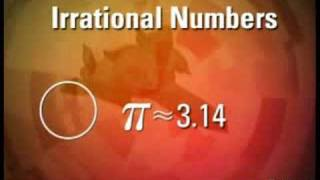By laneCrease

Discovery Education Video. Video discusses irrational numbers such as the number pi because it has no decimal equivalent. Then it discusses how irrational numbers relate to rational numbers. Video is good quality and good for all students as a review or initial learning of the topic.# Visualizing equivalent fractions | Fractions | Pre-Algebra | Khan Academy

##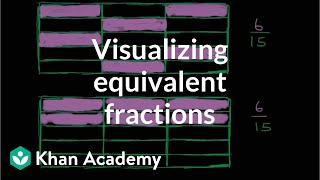By Khan Academy

To understand equivalent fractions it helps to see the fractions using something like a grid with shaded and unshaded sections representing the fraction.# Equivalent fractions and different wholes

##By Khan Academy

Learn how two fractions are equivalent only if they refer to the same whole. For example, 1/2 of a small circle is not the same as 1/2 of a big circle.# More on equivalent fractions | Fractions | 4th grade | Khan Academy

##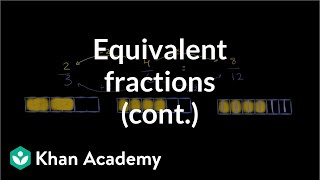By Khan Academy

Important: if you multiply a fraction's numerator and the denominator by the same number, you'll end up with an equivalent fraction. Got it? Maybe? No problem, we'll help!# Two-step equations with decimals and fractions

##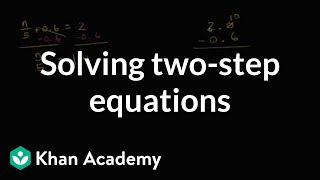By Khan Academy

Learn how to solve equations that involve decimals and fractions. The equations shown in this video are called two-step equations because they each take two steps to solve.# Equivalent fractions with visuals

##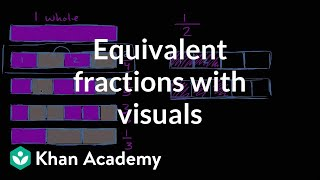By Khan Academy

Use same-sized wholes to show equivalent fractions.# One-step multiplication and division equations with fractions and decimals

##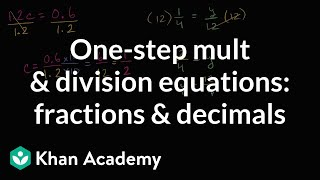By Khan Academy

Learn how to solve equations in one step by multiplying or dividing a number from both sides.ï¿½ï¿½ï¿½ï¿½ï¿½ï¿½ï¿½ï¿½ï¿½ï¿½ï¿½ï¿½These problems involve decimals and fractions.# One-step addition and subtraction equations with fractions and decimals

##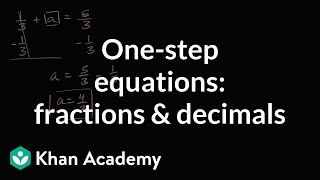By Khan Academy

Learn how to solve one-step addition and subtraction equations that have fractions and decimals in them.# One-step addition and subtraction equations with fractions and decimals

##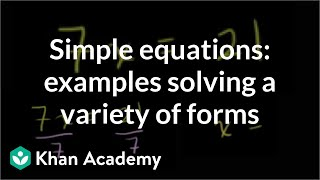By Khan Academy

Some quick examples to practice solving a variety of one step equations. All 4 operations (add, subtract, multiple, divide) are paired with variables.# One-step addition and subtraction equations with fractions and decimals

##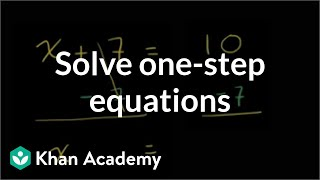By Khan Academy

Learn how to solve one-step addition and subtraction equations by adding or subtracting the same thing from both sides of the equation.# One-step addition and subtraction equations with fractions and decimals

##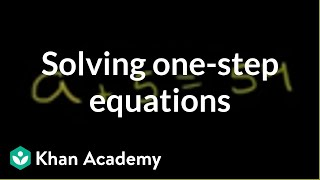By Khan Academy

Learn how to solve this equation: a + 5 = 54ï¿½ï¿½ï¿½ï¿½ï¿½ï¿½ï¿½ï¿½ï¿½ï¿½ï¿½ï¿½# One-Step Subtraction Equations - MathHelp.com - Math Help

##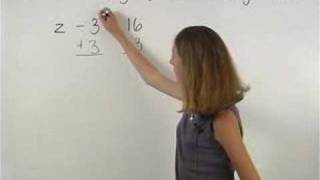By yourteachermathhelp

This video takes you through a one step subtraction equation and also shows you how to check your answer.# Sums and products of irrational numbers

##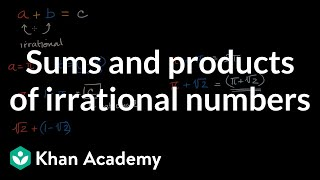By Khan Academy

Thinking about whether the sums and products of irrational numbers are rational or irrational.# One-step equations with multiplication and division

##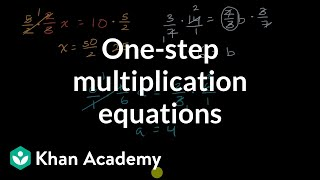By Khan Academy

Remember that what you do to one side, you have to do to the other. Will you multiply or divide both sides to dump the fraction, x/a? Let's do it together.# One-step equations with multiplication and division

##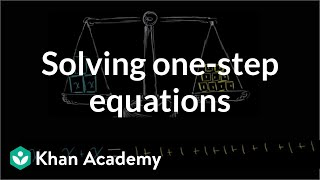By Khan Academy

This equation can be simplified through a single step to solve for the variable. Can you help?# One-step equations with multiplication and division

##By Khan Academy

Let's get a conceptual understanding of why one needs to divide both sides of an equation to solve for a variable.# One-step equations with multiplication and division

##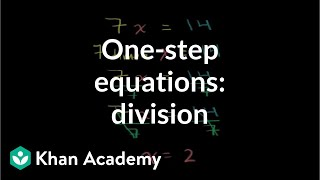By Khan Academy

Let's ease into this, shall we? Here's an introduction to basic algebraic equations of the form ax=b. Remember that you can check to see if you have the right answer by substituting it for the variable!# Fractions to Decimals | MathHelp.com

##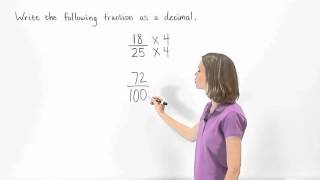By MathHelp.com# One-step equations with addition and subtraction

##By Khan Academy

Some quick examples to practice solving a variety of one step equations. All 4 operations (add, subtract, multiple, divide) are paired with variables.